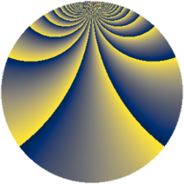# Properties

 Label 1323.2.zLevel $1323$ Weight $2$ Character orbit 1323.z Rep. character $\chi_{1323}(188,\cdot)$ Character field $\Q(\zeta_{14})$ Dimension $444$ Sturm bound $336$

# Related objects

## Defining parameters

 Level: $$N$$ $$=$$ $$1323 = 3^{3} \cdot 7^{2}$$ Weight: $$k$$ $$=$$ $$2$$ Character orbit: $$[\chi]$$ $$=$$ 1323.z (of order $$14$$ and degree $$6$$) Character conductor: $$\operatorname{cond}(\chi)$$ $$=$$ $$147$$ Character field: $$\Q(\zeta_{14})$$ Sturm bound: $$336$$

## Dimensions

The following table gives the dimensions of various subspaces of $$M_{2}(1323, [\chi])$$.

Total New Old
Modular forms 1044 444 600
Cusp forms 972 444 528
Eisenstein series 72 0 72

## Trace form

 $$444q + 72q^{4} + 3q^{7} + O(q^{10})$$ $$444q + 72q^{4} + 3q^{7} - 68q^{16} + 22q^{22} - 82q^{25} + 16q^{28} - 56q^{34} + 23q^{37} - 14q^{40} - 16q^{43} + 20q^{46} + 57q^{49} - 14q^{52} + 84q^{58} + 21q^{61} + 72q^{64} - 14q^{67} - 20q^{70} - 336q^{76} + 42q^{79} - 70q^{82} + 84q^{85} + 12q^{88} + 281q^{91} + 308q^{94} + O(q^{100})$$

## Decomposition of $$S_{2}^{\mathrm{new}}(1323, [\chi])$$ into newform subspaces

The newforms in this space have not yet been added to the LMFDB.

## Decomposition of $$S_{2}^{\mathrm{old}}(1323, [\chi])$$ into lower level spaces

$$S_{2}^{\mathrm{old}}(1323, [\chi]) \cong$$ $$S_{2}^{\mathrm{new}}(147, [\chi])$$$$^{\oplus 3}$$$$\oplus$$$$S_{2}^{\mathrm{new}}(441, [\chi])$$$$^{\oplus 2}$$